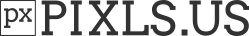# How to write clean G'MIC code ?

Lately, I’ve spent a lot of time “cleaning” the `.gmic` files located in `gmic-community/include` (i.e. G’MIC commands and filters written by others).
I’ve seen some rather strange stuffs, so I think it could be interesting to summarize some of the bad practices I’ve encountered, and explain how to make the code cleaner.
If you are not a G’MIC script writer, it probably won’t be of any interest to youLet’s start!

No particular example here, but of course, indenting code is a good practice to better understand the code, particularly when using nested conditions and loops.
Please don’t use the `TAB` character, as this never renders the same on different text editors.

### Testing a condition:

In G’MIC, the commands `if`, `elif`, `while` and `repeat` expect a math expression as an argument, and the argument will always be evaluated. Therefore, forcing the argument evaluation using braces `{expression}` is not necessary (and slower).
So,

``````if \$var1==\$value1" && "(\$var2==\$value2" || "\$var2==\$value3)
...
fi
``````

will work OK. No need for

``````if {\$var1==\$value1" && "(\$var2==\$value2" || "\$var2==\$value3)}`
``````

or, even worse (as I’ve seen it from times to times) :

``````if {{{\$var1==\$value1}" && "{{\$var2==\$value2}" || "{\$var2==\$value3}}}
``````

In the latter, the math expression evaluator is called 6 times instead of once!
(you have to remember that each call of the math evaluator mostly involves parsing an expression + compiling it into bytecode before evaluation…)

### Testing a variable against multiple values:

The G’MIC language has no specific syntax for that case, as we have in C/C++ with:
`switch(variable) { case value1: ... case value2: ... }`, so, basically we have two solutions for doing that:

1. Using `if...elif...elif...fi` :
``````if \$variable==0
# Do something
elif \$variable==1
# Do something else
elif \$variable==2
# Do something else
else
# Do something else, corresponds to the `default` case in C/C++.
fi
``````

Please think about using `elif`! I’ve seen quite bad things like:

``````if \$variable==0
# Do something
else
if \$variable==1
# Do something else
else
if \$variable==2
# Do something else
else
# Default case.
fi
fi
fi
``````

and this, sometimes without indentation!Definitely harder to read and understand.

1. Anyway, use the construction above only when you need to do very different stuffs for each case. For instance, if you just need to set a different value for a variable (e.g. the name of a command to perform colorspace conversion), then `\${arg\ ...}` is your friend, e.g.:
``````  convert_colors_fwd=\${arg\ 1+\$1,rgb2hsi,rgb2hsl,rgb2hsv,rgb2lab}
convert_colors_bwd=\${arg\ 1+\$1,hsi2rgb,hsl2rgb,hsv2rgb,lab2rgb}
\$convert_colors_fwd[-1]
# Do something...
\$convert_colors_bwd[-1]
``````

This will save you a lot of lines of code.

More to come2 Likes

I spy something that could help me with `csswap`

When you’re done, I plan to update Construction Material Texture. It’s broken right now, and is in need of a upgrade.

Improvement award to the both of you.@David_Tschumperle

There is no elif in case of fill or eval block. In that case, a good example for writing code is below. Hard to understand and manipulate at first though.

``````f "begin(
if(\$1<-1,
codeblock_1;,
if(\$1==-1,
codeblock_2;,
#if(\$1==0,#
codeblock_3;
);
);
);
valcode;
"
``````

I must say I’m not a big fan of the `if()` in math formulas.
I prefer to use the ternary operator `cond?then_expr:else_expr` that can be more easily pipelined to create things like `switch()... case.. ` blocks.
Like this:

``````f "begin(
\$1<1?(
codeblock_1;
):
\$1==1?(
code_block_2;
):( # Default
codeblock_default;
);
)"
``````
1 Like

@David_Tschumperle: Your test “\$1==-1” will never evaluate to true. Perhaps you intended “\$1<-1”, not “\$1<1”. (Or perhaps my brain has a bug.)

Not sure if you would add this section, but recently while reducing the number of lines in my codes. I noticed how to drastically reduce the line associated with permutations of numbers.

Based on my latest commit:

``````From:

if \$1==0 (0,1,2)
elif \$1==1 (0,2,1)
elif \$1==2 (1,0,2)
elif \$1==3 (1,2,0)
elif \$1==4 (2,0,1)
elif \$1==5 (2,1,0)
fi
channels={crop(#-1)}
gmic_function \$channels

To:
channel_order=[0,1,2,0,2,1,1,0,2,1,2,0,2,0,1,2,1,0]
order={\$1*3}
gmic_function {(\$channel_order)[\$order,3]}
``````

See the differences in number of codes?